Blog

# Functions and Examples of Generated Columns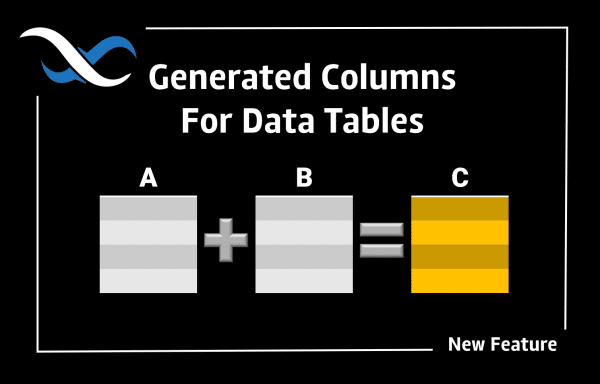One of the new features we added in Backendless is called “Generated columns”. If you are familiar with Excel and have ever used Excel cell functions, you will understand Backendless Generated columns in no time. In short, it is a special column you can declare in a data table that has a “formula”, or our lingo, an expression.

The expression is responsible for generating a value for the columns. What can you do in an expression? A LOT! And that’s what makes Backendless Generated Columns super powerful! Read this article for an overview of the new feature.

In this post, we will go over some examples so you can see how awesome this functionality is and can start using it for your application. Let’s start with something very basic.

## Basic Math

Suppose we have a table called `Math`. The table has two columns `operand1` and `operand2`, both are of the `INT` type.  Here’s the schema for the columns: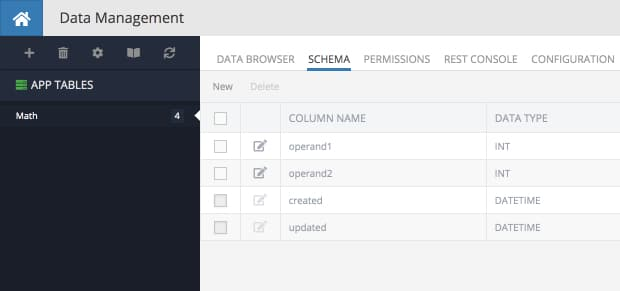Let’s say we want to be able to calculate the following values:

• the sum (`operand1 + operand2`)
• the difference (`operand - operand2`)
• the product (`operand1 * operand2`)
• the dividend (`operand1 / operand2`)
• `operand1` raised to the power of `operand2`, which is basically `(operand1)operand2`

And not only we want to calculate these values, but also have dedicated columns that contain each of the results. Generated columns to the rescue!

Here’s what a declaration of the column containing the sum would look like: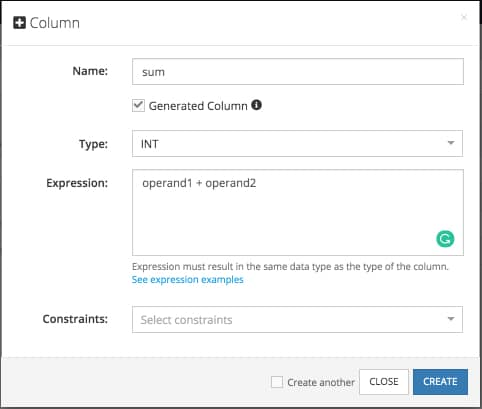As you can see all it takes is to select the Generated Column checkbox and then enter the Expression. Make sure to select the Type you expect the expression to produce. In our case, the sum of two integers will be an integer. The Expression field is the most important part. It is where you put the formula to calculate the column value. Once the column is created, let’s see it in action: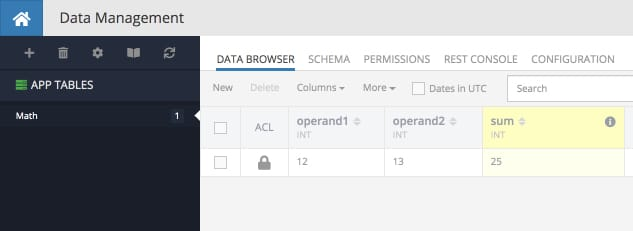As you can see the generated columns have a special visualization in Backendless Console – there is a light yellow background, which is meant to indicate that this is a special column. Indeed, you cannot enter values into the cells of that column and you cannot update that column’s values through the API. However, as you can see the result is right there – the column displays the value according to the provided expression – it is the sum of the `operand1` and the `operand2` values.

Let’s add more columns for other math operations. The subtraction, multiplication, and division are going to be easy since Backendless supports all basic math operations. But how to go about calculating the power of `operand1` to `operand2`? This is where expression functions come in. And we support a lot of them, including one to calculate the power (and Sine, Cosine, Tangent, Log, Square root, Random number generator to name a few). See below for the examples of what the expression should look like when you configure the generated columns the example we’re reviewing:

• difference – `operand - operand2`
•  product – `operand1 * operand2`
• dividend – `operand1 / operand2`
• power – `POWER( operand1, operand2 )`

This is what my data table looks like after I added the columns with the expressions shown above: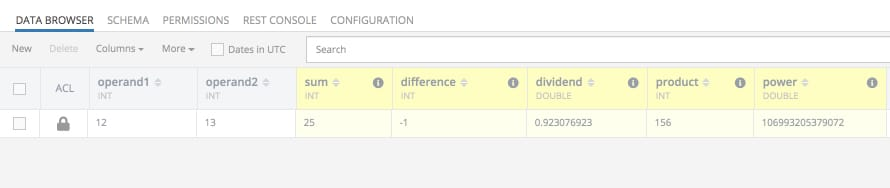Of course, being able to define a column that calculates the value according to your own formula/expression is fantastic, but what makes this infinitely more useful is the API handles the columns just like any other ones. What this means is:

• The where clause to retrieve data objects can reference generated columns;
• A generated column and its value is returned by the server as any other column;
• A generate column expression can reference other generated column;

Below is a list of some common mathematical functions you can use in your expressions. Where it says `value` it can be:

1. a scalar value
2. name of a column in the same table
3. another function whose result will be used as the input
• ABS( value ) – returns absolute value. Example:
Example: ```ABS( operand1 ) ```
• MOD( value1, value2 ) – Modulo operation. Returns the remainder of `value1` divided by `value2`.
Example: ```MOD( operand1, operand2 ) ```
• FLOOR( value ) -Returns the largest integer value not greater than `value`.
Example: ```FLOOR( operand1 ) ```
• RAND() -Returns a random floating-point value in the range 0 <= value < 1.0.To obtain a random integer R in the range i <= R < j, use the expression `FLOOR(i + RAND() * (j − i))`. For example, to obtain a random integer in the range 7 <= R < 12, use the following expression:
Example: `FLOOR(7 + (RAND() * 5))`

## Working with String Values

Suppose there is a table with two columns of the `STRING` type. The column names are `firstName` and `lastName`: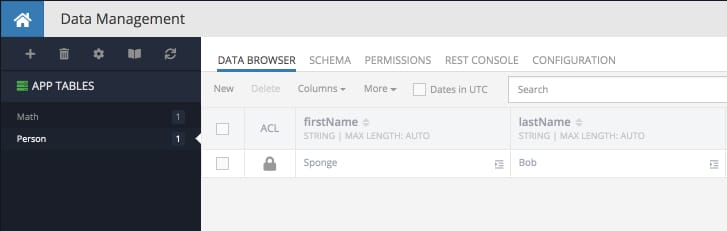Here are some examples of what you can do with generated columns and these string values:

• CONCAT( value1, value2, …, valueN ) – concatenate values.
Example: `CONCAT( firstName, ' ', lastName )` produces `"Spong Bob"`
• CONCAT_WS( separator, value1 value2, …, valueN ) – concatenate values with separator.
Example: `CONCAT_WS( ', ', firstName, lastName )` produces `"Sponge, Bob"`
• TO_BASE64( value ) – encodes value using the base-64 encoding.
Example: `TO_BASE64( firstName )` produces `U3Bvbmdl`
• FROM_BASE64( value ) – decodes a base-64 encoded value.
Example: `FROM_BASE64( TO_BASE64( firstName ) )` produces `"Sponge"`
• LENGTH( value ) – returns the length of value measured in bytes.
Example: `LENGTH( firstName )` produces 6
• LOWER( value ) – returns value in lower case.
Example: `LOWER( firstName )` produces `"sponge"`
• UPPER( value ) – returns value in upper case.
Example: `UPPER( CONCAT( firstName, ' ', lastName ) )` produces `"SPONGE BOB"`

There are many more date/time functions supported by Backendless. For a complete list, see the MySQL documentation.

## Working with Dates

Suppose there is a table with a column of type `DATETIME`.  The column name is `deliveryDate`: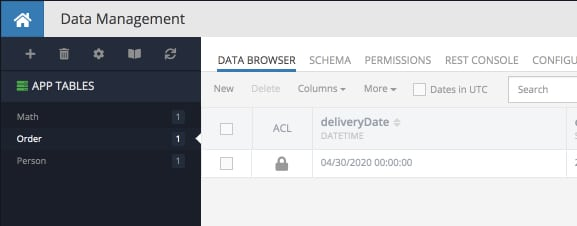Here are some examples of what you can do with generated columns and this DATETIME column:

• ADDDATE( value, days ) – adds the specified number of days to the value.
Example: `ADDDATE( deliveryDate, 15 )` returns `05/15/2020 00:00:00`
• DATEDIFF( value1, value2 ) – returns the difference between value1 and value2 where both values are of the DATETIME type.
Example: `DATEDIFF( created, deliveryDate )` – returns the number of days between the date when the order had been created and the delivery date.
• DAYNAME( value ) – returns the name of the weekday for value.
Example: `CONCAT( 'Your delivery will be on ', DAYNAME(deliveryDate) )` produces `"Your delivery will be on Thursday"`
• DAYOFMONTH( value ) – returns the day of the month for date, in the range 1 to 31.
Example: `DAYOFMONTH( deliveryDate )` produces 30
• DAYOFWEEK( value ) – returns the weekday index for date (1 = Sunday, 2 = Monday, …, 7 = Saturday)
Example: `DAYOFWEEK( deliveryDate )` produces 5
• DAYOFYEAR( value ) – returns the day of the year for date, in the range 1 to 366.
Example: `DAYOFYEAR( value )` produces 121
• HOUR( value ), MINUTE( value ), SECOND( value ) – return hour, minute and second elements of the datetime value. All elements are zero-based.

There are many more date/time functions supported by Backendless. For a complete list, see the MySQL documentation.

## Conditional Values (IF/THEN/ELSE)

It is possible to create a generated column with an expression that includes some conditional logic. Consider the following example, it is a table called `Order`.  Suppose we need to determine the priority of delivery depending on the day of the week of the delivery date. If the delivery date is between Monday through Friday, the priority is `"HIGH"` and if it is on the weekend (Saturday or Sunday), the priority is `"MEDIUM"`. Here’s what the expression for the generated column could look like:

```CASE
WHEN DAYOFWEEK( deliveryDate ) IN (2,3,4,5,6)
THEN 'HIGH'
WHEN DAYOFWEEK( deliveryDate ) IN (1,7)
THEN 'MEDIUM'
END```

When creating a generated column, the screen will look as shown below: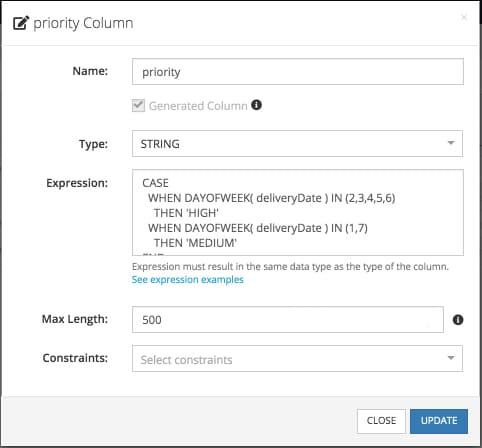And the magic happens automatically:

`"HIGH"` priority – the date lands on a weekday: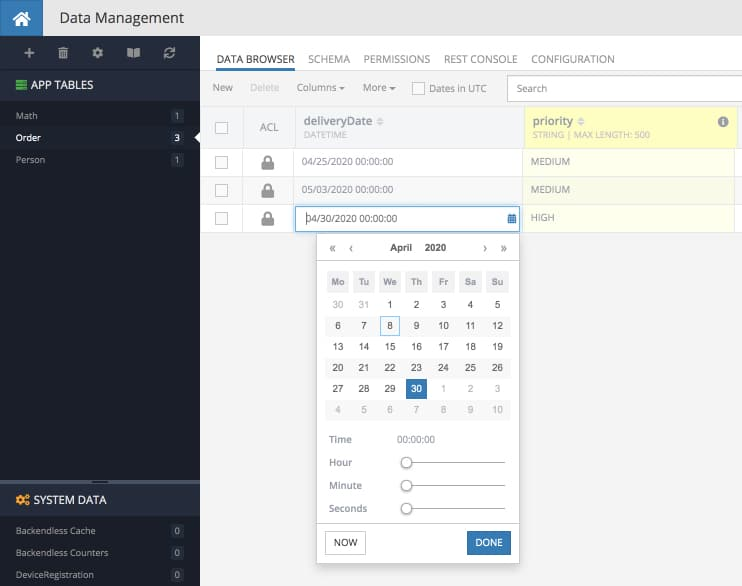`"MEDIUM"` priority – the date lands on the weekend: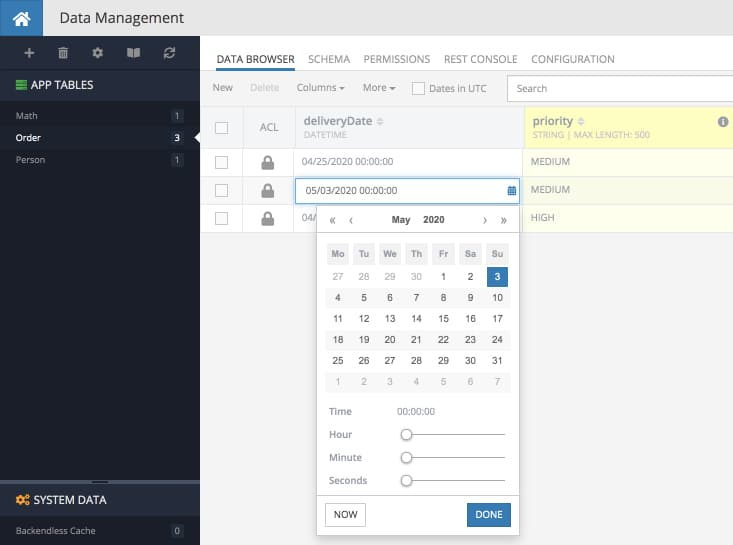Cheers and Happy Coding!